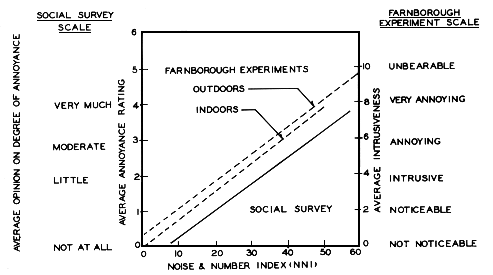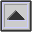NOISE AND NUMBER INDEX (NNI)

Originally devised by the Wilson Committee on Noise in Britain (1963), the Noise and Number Index is an attempt to measure the subjective noisiness of aircraft. It uses the PNdB as a basis and additionally takes into account the number of aircraft per day (or night) as a key annoyance factor. The NNI formula is as follows:

NNI = (Average Peak PNdB) + 15 (log10 N) - 80

where N is the number of aircraft, the average peak PNdB is a logarithmic average of the highest levels of all overflights, and 80 is the value subtracted to take into account the findings of social surveys which showed that the annoyance factor was zero at 80 PNdB (see graph below).

If the number of flights is known, PNdB may be converted to NNI quite easily. For instance, assuming an average peak of 105 PNdB (approx. 92 dBA) and the total number of flights to be 140, the conversion would be as follows:

NNI = 105 PNdB + 15 (log10 140) - 80
= 105 + 15 (2.146) - 80

= 105 + 32 - 80

NNI = 57

The Noise and Number Index scale runs from 0 - 60 and following the results of social surveys, the Wilson Committee assigned values of annoyance to the index as shown in the graph below. The Wilson Committee considered that exposure to aircraft noise reaches an unreasonable level in the range 50 - 60. A difference of 10 NNI corresponds either to an increase of the peak level of 10 PNdB or to a quadrupling of the number of flights in the period. Compare chart under COMMUNITY NOISE EQUIVALENT LEVEL. See also: SOUND INTRUSION.Relations between annoyance rating and Noise and Number Index obtained from social survey and Farnborough experiments. From: Wilson Committee on the Problem of Noise, Noise: Final Report, Cmnd 2056, London, HMSO, 1963, p. 208, used by permission.

home